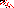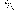# MINLPLib

### A Library of Mixed-Integer and Continuous Nonlinear Programming Instances

#### Instance: alkyl

 Formatsⓘ ams gms mod nl osil pip Primal Bounds (infeas ≤ 1e-08)ⓘ -1.76499965 p1 ( gdx sol ) (infeas: 3e-10) Other points (infeas > 1e-08)ⓘ -1.76502498 p2 ( gdx sol ) (infeas: 8e-07) Dual Boundsⓘ -1.76500127 (ANTIGONE)-1.76500008 (BARON)-1.76501147 (COUENNE)-1.76499966 (LINDO)-1.76500560 (SCIP) Referencesⓘ Berna, T J, Locke, M H, and Westerberg, A W, A New Approach to Optimization of Chemical Processes, American Institute of Chemical Engineers Journal, 26:1, 1980, 37-43. Sourceⓘ GAMS Model Library model alkyl Applicationⓘ Alkylation Added to libraryⓘ 31 Jul 2001 Problem typeⓘ NLP #Variablesⓘ 14 #Binary Variablesⓘ 0 #Integer Variablesⓘ 0 #Nonlinear Variablesⓘ 12 #Nonlinear Binary Variablesⓘ 0 #Nonlinear Integer Variablesⓘ 0 Objective Senseⓘ min Objective typeⓘ quadratic Objective curvatureⓘ indefinite #Nonzeros in Objectiveⓘ 6 #Nonlinear Nonzeros in Objectiveⓘ 2 #Constraintsⓘ 7 #Linear Constraintsⓘ 1 #Quadratic Constraintsⓘ 4 #Polynomial Constraintsⓘ 2 #Signomial Constraintsⓘ 0 #General Nonlinear Constraintsⓘ 0 Operands in Gen. Nonlin. Functionsⓘ Constraints curvatureⓘ indefinite #Nonzeros in Jacobianⓘ 25 #Nonlinear Nonzeros in Jacobianⓘ 17 #Nonzeros in (Upper-Left) Hessian of Lagrangianⓘ 21 #Nonzeros in Diagonal of Hessian of Lagrangianⓘ 1 #Blocks in Hessian of Lagrangianⓘ 3 Minimal blocksize in Hessian of Lagrangianⓘ 2 Maximal blocksize in Hessian of Lagrangianⓘ 8 Average blocksize in Hessian of Lagrangianⓘ 4.0 #Semicontinuitiesⓘ 0 #Nonlinear Semicontinuitiesⓘ 0 #SOS type 1ⓘ 0 #SOS type 2ⓘ 0 Infeasibility of initial pointⓘ 0.03 Sparsity JacobianⓘSparsity Hessian of Lagrangianⓘ```\$offlisting
*
*  Equation counts
*      Total        E        G        L        N        X        C        B
*          8        8        0        0        0        0        0        0
*
*  Variable counts
*                   x        b        i      s1s      s2s       sc       si
*      Total     cont   binary  integer     sos1     sos2    scont     sint
*         15       15        0        0        0        0        0        0
*  FX      0
*
*  Nonzero counts
*      Total    const       NL      DLL
*         32       13       19        0
*
*  Solve m using NLP minimizing objvar;

Variables  objvar,x2,x3,x4,x5,x6,x7,x8,x9,x10,x11,x12,x13,x14,x15;

Positive Variables  x2,x3,x4,x5,x6;

Equations  e1,e2,e3,e4,e5,e6,e7,e8;

e1.. 6.3*x5*x8 + objvar - 5.04*x2 - 0.35*x3 - x4 - 3.36*x6 =E= 0;

e2..  - 0.819672131147541*x2 + x5 - 0.819672131147541*x6 =E= 0;

e3.. 0.98*x4 - (0.01*x5*x10 + x4)*x7 =E= 0;

e4.. -x2*x9 + 10*x3 + x6 =E= 0;

e5.. x5*x12 - (1.12 + 0.13167*x9 - 0.0067*x9*x9)*x2 =E= 0;

e6.. x8*x13 - 0.01*(1.098*x9 - 0.038*x9*x9) - 0.325*x7 =E= 0.57425;

e7.. x10*x14 + 22.2*x11 =E= 35.82;

e8.. x11*x15 - 3*x8 =E= -1.33;

* set non-default bounds
x2.up = 2;
x3.up = 1.6;
x4.up = 1.2;
x5.up = 5;
x6.up = 2;
x7.lo = 0.85; x7.up = 0.93;
x8.lo = 0.9; x8.up = 0.95;
x9.lo = 3; x9.up = 12;
x10.lo = 1.2; x10.up = 4;
x11.lo = 1.45; x11.up = 1.62;
x12.lo = 0.99; x12.up = 1.01010101010101;
x13.lo = 0.99; x13.up = 1.01010101010101;
x14.lo = 0.9; x14.up = 1.11111111111111;
x15.lo = 0.99; x15.up = 1.01010101010101;

* set non-default levels
objvar.l = -0.9;
x2.l = 1.745;
x3.l = 1.2;
x4.l = 1.1;
x5.l = 3.048;
x6.l = 1.974;
x7.l = 0.893;
x8.l = 0.928;
x9.l = 8;
x10.l = 3.6;
x12.l = 1;
x13.l = 1;
x14.l = 1;
x15.l = 1;

Model m / all /;

m.limrow=0; m.limcol=0;
m.tolproj=0.0;

\$if NOT '%gams.u1%' == '' \$include '%gams.u1%'

\$if not set NLP \$set NLP NLP
Solve m using %NLP% minimizing objvar;

```

Last updated: 2021-03-11 Git hash: 66559cbc